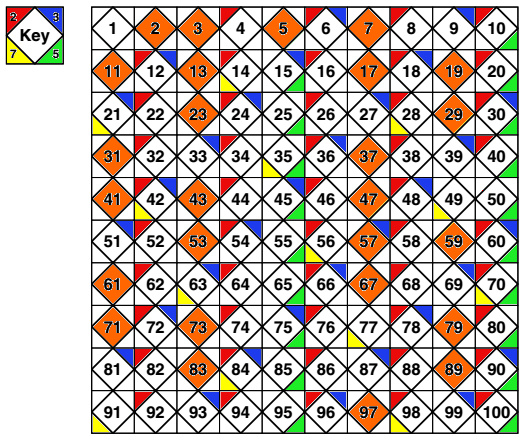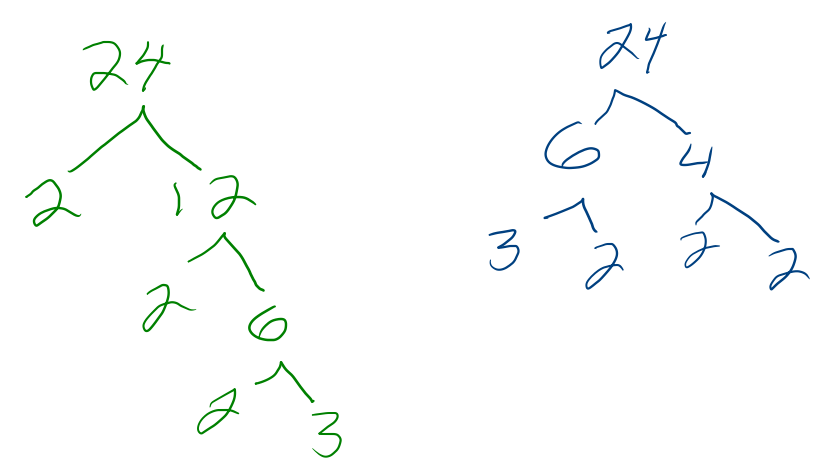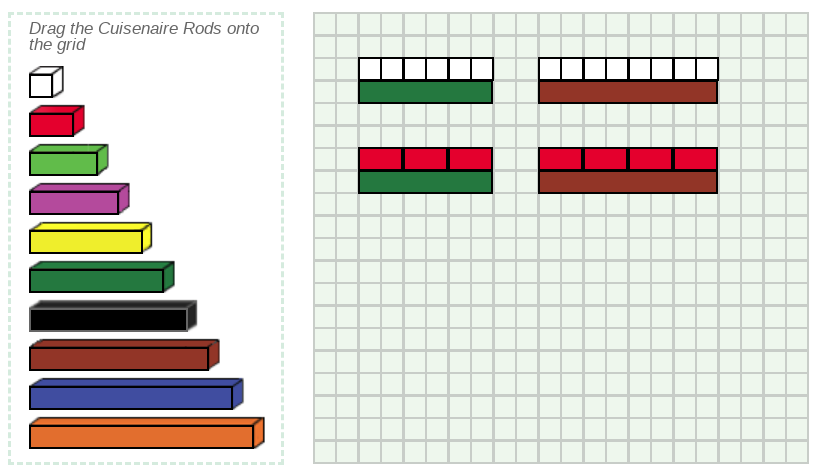MTH212
Notes on Number Theory and Fractions
Winter 2019

### Chapter 4 Number Theory

#### § 4-1 Divisibility

b divides a, b|a, if and only if, there is a q such that a=b*q.

for example

1. 2|6 since 6=2*3
2. 6 cancel{|} 2
3. 0|2
4. 2|4n for all whole numbers n
##### Divisibility Rules
• If d | a and d | b, then d | (a+b).
Ex: 3|6 and 3|9, so 3|15
• If d | a and d cancel{|} b, then d cancel{|} (a+b).
Ex: 3|6 and 3 cancel{|} 10, so 3 cancel{|} 16
• If d | a and d | b and a>=b, then d | (a-b).
• If d | a and d cancel{|} b and a>=b, then d cancel{|} (a-b).
• If d cancel{|} a and d | b and a>=b, then d cancel{|} (a-b).
##### Divisibility Tests
2
the ones digit is a 0,2,4,6,8
3
the sum of the digit is a multiple of 3
4
the two right-most digits form a multiple of 4
5
the ones digit is a 0,5
6
meets the tests for 2 and 3
8
the three right-most digits form a multiple of 8
9
the sum of the digit is a multiple of 9
10
the ones digit is a 0
11
the difference between the sum of the digits from the even powers of 10 and the sum of the digits from the odd powers of 10, is a multiple of 3

#### § 4-2 Primes and Composites

Consider the image below:The corners show divisibility by 2, 3, 5, and 7. Numbers with any colored corners are composite. Orange numbers are prime.

##### Terminology
Divisor
a number that is a factor of another number
b divides a, b|a, if and only if, there is a q such that a=b*q.
Factor
a number that is a divisor of another number
Multiple
the product of a given number with any whole number
Prime
exactly two distinct divisors
Composite
more than two distinct divisors
Factorization
write as a product of whole numbers
Prime Factorization
write as a product of only prime numbers
Factor Trees
factor a number as a pair of factors; repeat with each factor until only prime numbers remain.#### § 4-3 Greatest Common Divisor and Lowest Common Multiple

##### GCD methods
###### The List Method:
Consider 24 and 18:
List the factors of each number.
24: 1, 2, 3, 4, 6, 8, 12, 24
18: 1, 2, 3, 6, 9, 18
Identify the common factors.
1, 2, 3, 6
6 is the greatest of the common factors.
###### The Color Rod Method:###### The Prime Factorization Method:
Consider 24 and 18:
Find the prime factorization of each number.
24 = 2^3*3
18 = 2*3^2
Select the lowest power of each common prime to multiply.
2*3 =6
###### The Euclidean Algorithm:
Consider 24 and 18:
Repeated division of a sort
24 = 18*1+6
18 = 6*3+0
SOnce you get a zero remainder, the previous remainder must be the GCF.
GCF =6